## Implicit Function Theorem

Givenif the Jacobian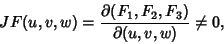then,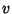, andcan be solved for in terms of,, andand Partial Derivatives of,,with respect to,, andcan be found by differentiating implicitly.

More generally, letbe an Open Set inand letbe aFunction. Write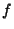in the form, whereandare elements ofand. Suppose that (,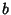) is a point insuch that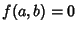and the Determinant of theMatrix whose elements are the Derivatives of thecomponent Functions ofwith respect to thevariables, written as, evaluated at, is not equal to zero. The latter may be rewritten asThen there exists a Neighborhoodofinand a uniqueFunctionsuch thatandfor all.